# Chemical Bonding and Molecular Structure# Chemical Bonding and Molecular Structure

Chemical Bonding and Molecular Structure

It is observed that the atoms of all the elements, except those for noble gases, tend to remain in a combined state with the atoms of the same or other elements. They do not exist as single atoms under ordinary conditions. Such atomic aggregates occur as molecules.

# Properties of Ionic Bond and Octet Rule:

 The attractive force that binds the atoms together in a molecule is called a chemical bond. It is formed either by the transfer of electrons or by the sharing of electrons.

The elements with one, two, three, four, ﬁve, six, or seven electrons in the outer shell, use these electrons to complete octet. The electrons which take part in two or more atoms to complete an octet is known as Electrovalency.

Lewis Dot Structure:  It is a shorthand to represent the valence electrons of an atom. The structures are written as the element symbol surrounded by dots that represent the valence electrons. The inner electrons are well protected and they are called core electrons.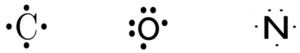Octet Rule: This rule was proposed by Lewis and Kossel. According to this rule, atoms undergo chemical reactions in order to attain an octet of electrons in the valence shell. Or, atoms containing 8 electrons in their valence shell are stable. To attain octets, they share electrons or exchange electrons. Furthermore, the atom does this by bonding with each other. Moreover, these atoms can be the same element or with different elements.
Two notable exceptions to the octet rule are helium and hydrogen. This is because both are happy with two electrons belonging to the outer shells.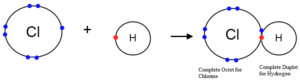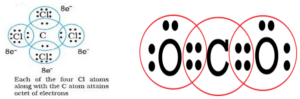Limitations of octet rule:
Formation of electron-deficient compounds like BeCl2, BF3, AlCl3. Central atom has less than 8e.
Formation of hypervalent compounds like PCl5, SF6, IF7, H2SO4 in which the central atom has more than 8 electrons.
Formation of compounds of noble gases like XeF2, XeF4, XeF6.
Odd electron molecules like NO, NO2.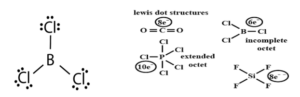Types of Chemical Bonds: The chemical bonds are classified into the following types

• Ionic bond
• Covalent bond
• Dative bond or Co-ordinate bond
• Hydrogen bond
• Metallic bond

Ionic bond: A bond formed by the transfer of an electron between 2 atoms is called an ionic or electrovalent bond. Or, it is the force of attraction between two oppositely charged ions. These ions are held together by the electrostatic force of attraction. The number of electrons lost or gained is known as electro-valency.
Na          →    Na+ +e
2,8,1               2,8
Cl + e    →    Cl
2,8,7               2,8,8
Na+ + Cl  → NaCl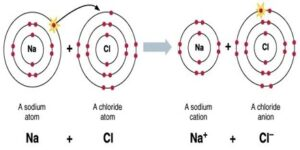Let us take another example of MgCl2 (Magnesium Chloride):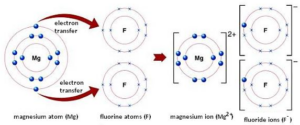Energy changes during the formation of an Ionic Bond:
An ionic bond formation involves the following steps:
The formation of a positive ion (cation) from the electropositive atom. The amount of energy required for this process is called ionization enthalpy (∆iH).
M(g) + ∆iH   →    M+(g) + e
Formation of negative ion from the electronegative atom. The energy involved in this process is called electron gain enthalpy (∆egH).
X(g) + e      →      X(g) + ∆egH
The packing of the cation and anion to form an ionic compound. The energy change in this process is called lattice enthalpy (∆latticeH)
M+ + X        →      MX(s) + ∆latticeH

Factors favouring the formation of ionic bonds:
Low ionization enthalpy (IE)
More negative electron gain enthalpy (EGE)
High lattice enthalpy (LE) If LE + EGE > IE, an ionic bond is formed.

Properties of ionic compounds:
Non-directional nature of ionic bond: These compounds do not exist as individual molecules. Instead, they are often hard solids, always made up of ions held together by strong electrostatic forces of attraction between ions with opposite charges.

Electrical conductivity: When added to water, the ions separate. The solution can thus conduct electricity. Similarly, these compounds conduct electricity in the fused state also.

Melting and boiling points: These compounds generally have high melting points. This is because a lot of thermal energy is required to break down the inter-ionic forces and form a liquid. Similarly, they possess high boiling points.

Solubility: These compounds are usually soluble in water but insoluble in benzene or other organic solvents.

Chemical Bonding and Molecular Structure

Covalent Bond: When a bond is formed by the sharing of electrons by the two atoms the bond formed between them is called a covalent bond. Each pair of shared electrons is indicated by a line -. Once the covalent bond has been formed, the two bonding electrons are attracted by the two nuclei instead of one, so the bonded state becomes more stable than the non-bonded state.
For eg. The formation of a Chlorine molecule (Cl2)
17Cl      +     17Cl    →   Cl2
2,8,7              2,8,7
Both the chlorine atoms have 7 electrons in their outermost shell. So here the exchange of electrons is not possible. For the formation of a Cl2 molecule, each Cl atom shares one pair of electron. The bond thus formed is called a single covalent bond.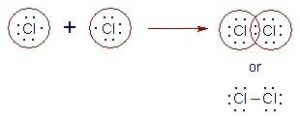The formation of an O2 molecule
8O       +    8O      →     O2
2,6            2,6
To attain an octet, each oxygen atom shares 2 pairs of electrons. Thus, a double covalent bond is formed.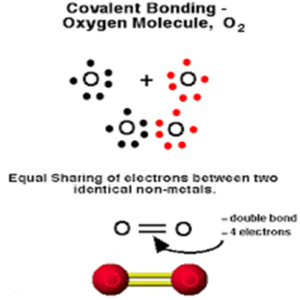The formation of N2 molecule
7N       +    7N      →     N2
2,5             2,5
To attain the octet configuration, the nitrogen atoms share 3 pairs of electrons. Thus a triple bond is formed.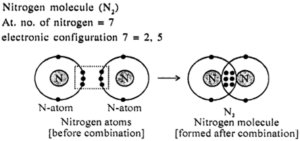The number of covalent bonds formed by an atom is termed its covalency. In the formation of the Cl2 molecule, the covalency of each Cl atom is one, in the O2 molecule, the covalency of O atom is 2 and in N2, it is 3. The double and triple bonds are together called multiple bonds.

Properties of Covalent Compounds: The covalent compounds are found to possess the following general properties, which are opposite to those of ionic compounds:
(a) These substances are made up of individual covalent molecules with weak intermolecular forces.
(b) Because of the weak intermolecular forces, the substances are gases, liquids, or soft solids at room temperature.
(c) These substances when put into the water do not undergo ionization. Hence these substances in the fused state or in solution do not conduct electricity. However, in presence of water, some polar covalent compounds can ionize and conduct electricity.
(d) These substances have low melting and boiling points because less energy is needed to break down the weak intermolecular forces.
(e) These substances are commonly soluble in benzene and other organic solvents but insoluble in water.

Chemical Bonding and Molecular Structure

Resonance Dipole Moment and Bond Parameters

Covalent Bond Parameters:
(1) Bond Length: It is defined as the distance between the nuclei of two bonded atoms in a molecule. It is measured by spectroscopic, X-ray diffraction, and electron diffraction techniques. It is expressed in pico-meter (pm) or in the angstrom unit (A0). Bond lengths depend upon the relative sizes of the atoms. For example, the bond lengths of hydrogen halides follow the sequence H – F < H – Cl < H – Br < H – I because the size of the halogens increases down the periodic group as F < Cl < Br < I. In the case of atoms joined by multiple bonds, the greater is the bond order, the smaller will be the bond length.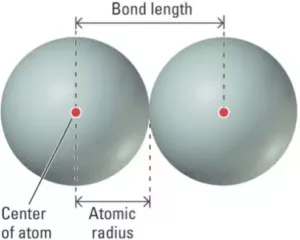(2) Bond Angle: It is defined as the angle between the orbitals containing bonding electron pairs around the central atom in a molecule. It can be experimentally determined by spectroscopic methods. It is expressed in degree.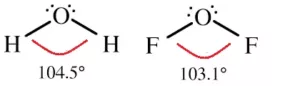(3) Bond Enthalpy (Bond Energy): Energy is released during the process of covalent bond formation between atoms which leads to the lowering of energy and energy is required to break a bond. Bond enthalpy (or bond energy) is defined as the amount of energy required to break one mole of a particular bond between 2 atoms in a gaseous state. Its unit is kJ/mol.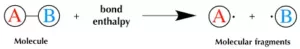(4) Bond Order: The bond order is the number of bonds present between two atoms in a molecule or ion. The bond order is said to be 1 when there is only one covalent bond formed between two atoms. For example Bond order in Cl2 and H2 is 1,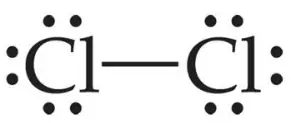Bond order for O2 is 2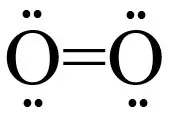Generally, with an increase in bond order, bond enthalpy increases and bond length decreases.

Dative bond or Co-ordinate bond: When in the formation of a bond between two atoms, only one atom contributes the pair of electrons and the other atom simply participates in sharing, the bond formed is called a dative bond or Co-ordinate bond. This may be explained as under:
In this case, one atom has its octet complete and has at least one lone pair (unshared) of electrons. The lone pair of electrons belonging to the atom with the complete octet is shared with the other atom containing an empty orbital and thus a link is established. The atom contributing the shared pair of electrons is called the donor while the atom which accepts these shared electrons is called the acceptor. This linkage set up between two atoms is represented by an arrow starting from the donor to the acceptor.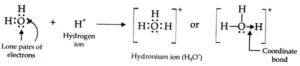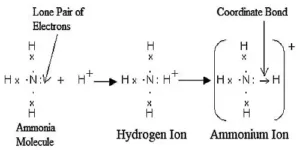Resonance and Resonating Structure: In the case of some compounds, all the observed properties cannot be explained by a single structure so we use more than one structure of the compound. These different structures are called resonance structures or canonical structures or contributing structures. The phenomenon is known as resonance. The contributing structures are written by keeping the following points in mind.
(i) In each contributing structure, the position of atoms should be the same, only the arrangement of electrons may change.
(ii) Each structure must have the same number of unpaired electrons.
(iii) The contributing structures should have energies close to each other. The contribution of a less stable contributing structure is also less.
(iv) A more stable contributing structure is one in which the negative charge resides on the most electronegative atom and a positive charge on the most electropositive atom.
(v) Various contributing structures are written with a double-headed arrow between them.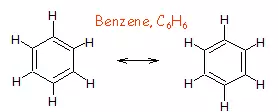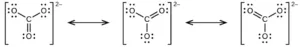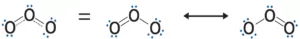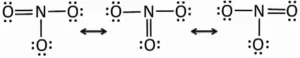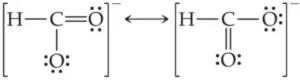Characteristics of Resonance:
(i) Resonance stabilizes the molecule. As the number of resonating structures increases, the stability also increases.
(ii) Resonance changes the bond length.
(iii) The difference in energy between the actual structure and the most stable canonical structure is called resonance energy. The greater the resonance energy, the stabler will be the molecule.

Chemical Bonding and Molecular Structure

The polarity of Bonds:
(i) Non–Polar Covalent Bond: If two similar atoms come close to each other and form a bond by sharing their electrons the shared electrons are equally attracted by the atoms as their electronegativity is the same. Hence, no poles are developed. eg. Cl2, N2, O2, H2.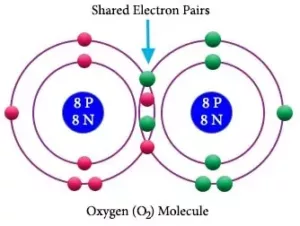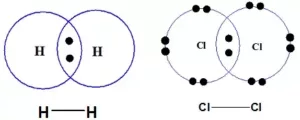(ii) Polar Covalent Bond: When two dissimilar atoms combine together to form a covalent bond, the shared pair of electrons shifts towards an atom having high electronegativity. As a result, more electronegative atom acquires a slight negative charge (δ) and the other gets a slightly positive charge (δ+). Such molecules are called polar molecules. E.g. HCl, HF, H2O, HI, etc.
The polar character of covalent bonds is measured with the help of a property called dipole moment (μ). It is defined as the product of the magnitude of charge (q) and the distance (d) between the two atoms.                             μ  =  q  x  d   Debye
The unit of dipole moment is Coulomb metre (Cm). But it is usually expressed in the unit Debye (D). 1D = 3.336 x 10-30 Cm. The dipole moment is a vector quantity. i.e. it has both magnitude and direction. It is represented by the arrow pointing towards the negative end. For eg.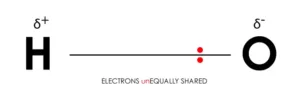In the case of polyatomic molecules, dipole moment depends on the individual bond dipoles and the spatial arrangement of bonds. Here the dipole moment of the molecule is the vector sum of the bond dipoles of various bonds.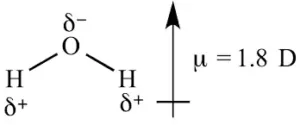For BeF2, the net dipole moment is zero, since the two equal bond dipoles are in opposite directions and cancel each other.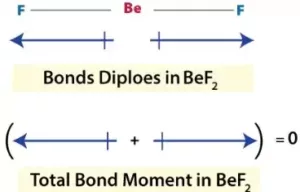In BF3, the net dipole moment is zero. Here the resultant of any 2 bond dipoles is equal and opposite to the third.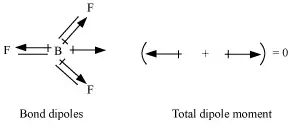Both ammonia (NH3) and nitrogen fluoride (NF3) are pyramidals in shape. Even though F is more electronegative than H, the net dipole moment of NF3 is smaller than that of NH3. This is because, in the case of NH3, the orbital dipole due to the lone pair is in the same direction as the resultant dipole moment of the three N – H bonds. But in NF3, the orbital dipole is in the opposite direction to the resultant dipole moment of the three N-F bonds. So, the dipole moments get partially canceled.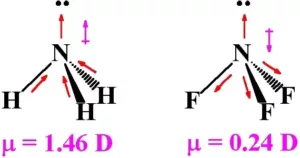Covalent Character in Ionic Compounds (Fajan’s Rule):
(i) There is no compound that is 100% ionic.
(ii) Covalent character in the ionic compound can be explained with the help of Fajan’s rule.
(iii) The smaller the size of the cation and the larger the size of the anion, the greater the covalent character of an ionic bond.
(iv) The greater the charge on the cation, the greater the covalent character of the ionic bond.
(v) (n-1)dnns0 is more polarising than the ion with a noble gas configuration (ns2 np6).

Chemical Bonding and Molecular Structure

Valance Electron Theories and Orbital Overlap

The valence shell electron pair repulsion (VSEPR) Theory:
This theory was proposed by Sidgwick and Powell and later modified by Nyholm and Gillespie. The important postulates of this theory are:
(i) The shape of a molecule depends upon the number of valence shell electron pairs (bonded or nonbonded) around the central atom.
(ii) Pairs of electrons in the valence shell repel one another since their electron clouds are negatively charged.
(iii) These pairs of electrons tend to occupy such positions in space that minimize repulsion and thus maximize the distance between them.
(iv) The valence shell is taken as a sphere with the electron pairs localizing on the spherical surface at maximum distance from one another.
(v) A multiple bond is treated as if it is a single electron pair and the two or three electron pairs of multiple bonds are treated as a single super pair.
(vi) Where two or more resonance structures can represent a molecule, the VSEPR model is applicable to any such structure.
(vii) The repulsive interaction of electron pairs decreases in the order: Lone pair (lp) – Lone pair (lp) > Lone pair (lp) – Bond pair (bp) > Bond pair (bp) –Bond pair (bp)

Shapes of the Molecules or Ions with Bond Pairs of electrons on the Central Atom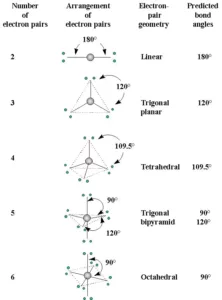Shapes of the Molecules or Ions with Lone Pairs and Bond Pairs of electrons on the Central Atom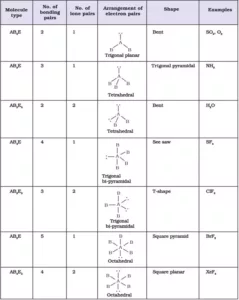Explanation of shapes of Molecules NH3 and H2O:
NH3: In ammonia, the central atom N has 5 valence electrons (7N – 2,5). Among these electrons, three are used for the formation of bonds with hydrogen atoms and the remaining 2 electrons stay as lone pairs. So, there are 4 VSEPs. Hence the expected shape of the molecule is tetrahedral. But due to the presence of lone pairs, the shape is distorted to trigonal bipyramidal, and the bond angle changes from 109028l to 1070.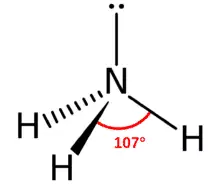H2O: In water, the central atom O has 6 valence electrons (8O – 2,6). Two of them are used for the formation of bonds with hydrogen atoms and the remaining 4 electrons stay as lone pairs. So there are 4 VSEPs. Hence the expected shape of the molecule is tetrahedral. But due to the presence of 2 lone pairs, the shape is distorted bent or angular or inverted v shape, and the bond angle changes from 109028l to 104.50.

Formal Charge: A formal charge (FC) is the charge assigned to an atom in a molecule. When determining the best Lewis Structure(or predominant resonance) for a molecule, the structure is chosen such that the formal charge on each of the atoms is as close to zero as possible.
Formula to Calculate Formal Charge: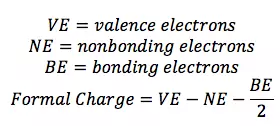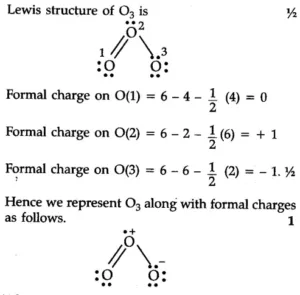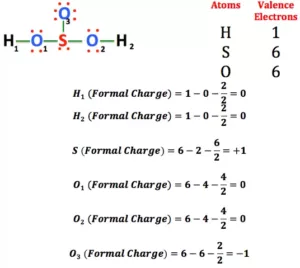Uses of Formal Charge:
1. The formal charge being a theoretical charge doesn’t indicate any real charge separation in the molecule.
2. Formal charges help in the selection of the lowest energy structure from a number of possible Lewis structures for a given species.
3. Knowledge of the lowest energy structure helps in predicting the major product of a reaction and also describes a lot of phenomena.
4. Generally, the lowest energy structure is the one with the smallest formal charges on the atoms and the most distributed charge.

Chemical Bonding and Molecular Structure

Valence Bond Theory: This theory was introduced by Heitler and London and later developed by Linus Pauling in order to explain the shape of molecules theoretically. This theory can be described in the following points.
(i) A covalent bond is formed when the orbital of one atom is situated in such a way that it overlaps with the orbital of another atom, each of them containing one unpaired electron.
(ii) The atomic orbitals overlap and the overlapped region is occupied by both the electrons. These two electrons must have opposite spins .
(iii) As a result of this overlapping, there is a maximum electron density between the two atoms. A large part of the binding force of the covalent bond results from the attraction of these electrons by the nuclei of both atoms.
(iv) Atoms maintain their individuality. When the bond is formed, only valence electrons from each bonded atom are involved and the inner atomic orbitals of each atom remain undisturbed.
Consider 2 hydrogen atoms A and B with nucleus NA and NB and electrons eA and eB respectively. When the two atoms are at a large distance from each other, there is no further interaction between them. Due to this their potential energy is zero. When the two atoms start approaching each other, new attractive and repulsive forces begin to operate.
The attractive forces arise between the nucleus of one atom and the electron of another atom i.e., NA– eB, and NB– eA. Similarly, repulsive forces arise between electrons of two atoms i.e., eA – eB, and the nuclei of two atoms NA – NB. Attractive forces bring the two atoms close to each other whereas repulsive forces push them away.
The magnitude of the new attractive force is more than the new repulsive forces. So, the two atoms approach each other and potential energy decreases. At a particular stage, the net attractive force balances the net repulsive forces and the energy becomes minimum. At this stage, the hydrogen atoms are said to be bonded together to form a stable molecule.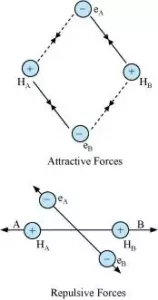Let us discuss another example of the formation of He2 molecule in which the number of attractive forces are less in comparison to the number of repulsive interactions so the He2 molecule does not exist.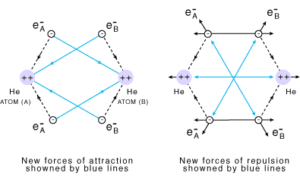Orbital Overlap Concept: The overlapping of the atomic orbital is the process of the partial interpenetration of atomic orbitals. The important characteristics of orbital overlapping are:
1. A covalent bond is formed by the overlapping of half-filled atomic orbitals present in the valence shell of atoms
2. The overlapping orbitals should contain an electron with opposite spin
3. Due to overlapping, the electrons get paired and a stable covalent bond is formed
4. The strength of a covalent bond depends on the extent of overlapping. The greater the extent of overlapping, the stronger will be the covalent bond formed

Types of Overlapping: There are two types of orbital overlapping.

Axial Overlapping or Sigma Bond: This type of covalent bond is formed by the end to end (hand-on) overlap of bonding orbitals along the internuclear axis. This is called head-on overlap or axial overlap. All single bonds are sigma bonds. A sigma bond can be formed in the following ways:
(i) s-s overlapping: Here the overlapping of two half-filled s-orbitals takes place along the internuclear axis.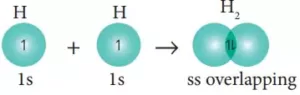(ii) s-p overlapping: Here the overlapping of half-filled s-orbitals and incomplete p-orbital take place along with the internuclear axis.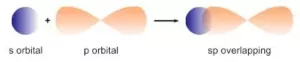(iii) p-p overlapping: Here two half-filled p- orbitals of two atoms overlap.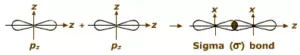pi(π ) bond: In the formation of the π bond the atomic orbitals overlap in such a way that their axes remain parallel to each other and perpendicular to the internuclear axis or bond formed by sidewise overlapping of atomic orbitals called a pi bond. The orbitals formed due to sidewise overlapping consist of two charged clouds above and below the plane of the participating atoms.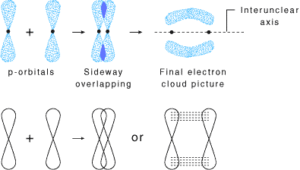A π bond is always present along with σ bonds. A double bond contains one σ bond and one π bond. A triple bond contains one sigma bond and two pi bonds.

A sigma bond is stronger than a pi bond. This is because the extent of overlapping is greater in a sigma bond.

Difference between Sigma and Pi Bond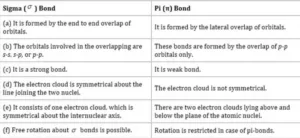Chemical Bonding and Molecular Structure

Concept of Hybridisation with Examples

Hybridization: It can be defined as the process of intermixing of the orbitals of slightly different energies so as to redistribute their energies, resulting in the formation of a new set of orbitals of equivalent energies and shape.

Salient Features of hybridization:
(i) The number of hybrid orbitals is equal to the number of atomic orbitals that get hybridized.
(ii) The hybridized orbitals are always equivalent in energy and shape.
(iii) The hybrid orbitals are more effective in forming stable bonds than the pure atomic orbitals.
(iv) These hybrid orbitals are directed in space in some preferred direction to have minimum repulsion between electron pairs and thus a stable arrangement.

Conditions of hybridisation:
(i) The orbitals present in the valence shell of the atom are hybridized.
(ii) The orbitals undergoing hybridization should have almost equal energy.
(iii) Promotion of electrons is not an essential condition before hybridization.
(iv) Completely filled orbitals of valence shells can also take part in hybridization.

 Type of Hybridization Orbitals involved Arrangement of hybrid orbitals sp One s and one p Linear sp2 One s and two p Trigonal planer sp3 One s and three p Tetrahedral sp3d One s, three p, and one d Trigonal bipyramidal sp3d2 or d2sp3 One s, three p, and two d Octahedral or Square bipyramidal sp3d3 One s, three p, and three d Pentagonal bipyramidal

sp Hybridisation: This type of hybridization involves the mixing of one s and one p orbital resulting in the formation of two equivalent sp hybrid orbitals. Each sp hybrid orbitals has 50% s-character and 50% p-character. Such a molecule in which the central atom is sp-hybridized and linked directly to two other central atoms possesses linear geometry. For example

(i) BeCl2 molecule forms in the following way: In BeCl2, the central atom Be has the electronic configuration 1s22s2. In the excited state, one of the 2s electrons is promoted to the 2p level. So, the configuration becomes 1s22s12p1. Now one s-orbital and one p-orbital undergo sp hybridization to form 2 new sp hybrid orbitals. Each of these hybrid orbitals overlaps with the 2p orbitals of Cl to form 2 Be-Cl bonds. So the shape of the molecule is linear with a bond angle of 1800.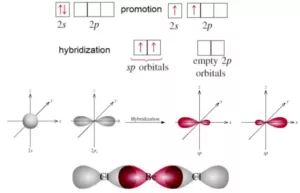(ii) Formation of Ethyne or Acetylene (C­2H2): In acetylene, each C atom undergoes sp hybridization. Out of the 2 sp hybrid orbitals, one of each C overlaps axially to form a C-C σ bond. The remaining two sp hybrid orbitals of each C overlap with the 1s orbital of H to form 2 C-H σ bonds.
Now each C atom has 2 unhybridized p-orbitals, which overlap laterally to form 2 π bonds. Thus, the molecule has a linear shape with a bond angle of 1800. Here there are 3 σ bonds and 2 π bonds in ethyne.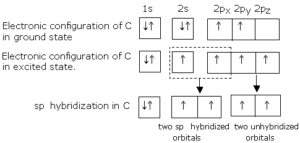sp2 Hybridisation: In this hybridization, there is the involvement of one s and two p-orbitals in order to form three equivalent sp2 hybridized orbitals. Each sp2 hybrid orbitals has 33.33% s-character and 66.66 % p-character. Such a molecule in which the central atom is sp2 hybridized and linked directly to three other central atoms possesses trigonal planar geometry. For example
(i) Formation of BCl3 molecule: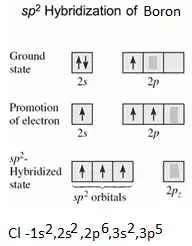sp3 Hybridisation: In this type of hybridization there is the mixing of one s-orbital and three p-orbitals of the valence shell to form four sp3 hybrid orbitals of equivalent energies and shape. There is 25% s-character and 75% p character in each sp3 hybrid orbital. The four sp3 hybrid orbitals so formed are directed towards the four corners of the tetrahedron. For Example:
(i) Formation of Methane (CH4): In CH4, the central atom C has the electronic configuration 6C – 1s22s22p2.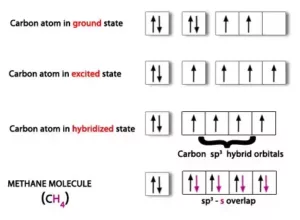(ii) Formation of Ammonia (NH3) Molecule: In NH3, the central atom N has the electronic configuration 1s22s22p3.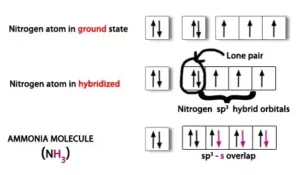The one s-orbital and three p-orbitals of N undergo sp3 hybridization to form 4 sp3 hybrid orbitals. One of these sp3 hybrid orbital is occupied by a lone pair and the other three sp3 hybrid orbitals overlap with 1s orbital of hydrogen to form 3 N-H bonds. Due to the greater repulsion between lone pair and bond pairs, the shape is distorted to pyramidal and the bond angle becomes 1070.

sp3d Hybridisation: It is the process of intermixing of one s-orbital, three p-orbitals, and one d-orbital to form five new orbitals having equivalent energy and shape. The 5 new orbitals formed are called sp3d hybrid orbitals. These are directed to the five corners of a regular trigonal bipyramid with bond angles 1200 and 900.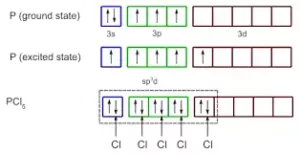In PCl5, three P-Cl bonds lie in one plane, at an angle of 1200. These three bonds are called equatorial bonds. The other two P-Cl bonds lie one above and one below this plane. They are called axial bonds. The axial bond pairs suffer more repulsion from the equatorial bond pairs. So, the axial bond length is greater than the equatorial bond length. So PCl5 is highly unstable and is very reactive.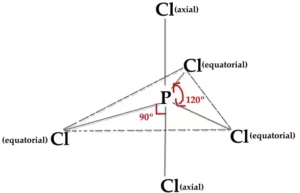sp3d2 Hybridisation: It is the process of intermixing of one s-orbital, three p-orbitals, and two d-orbitals to form six new orbitals having equivalent energy and shape. The 6 new orbitals formed are called sp3d2 hybrid orbitals. These are directed to the six corners of a regular octahedron with a bond angle 900. For example, the formation of SF6.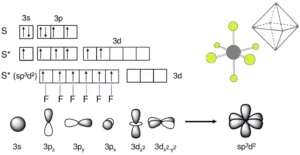Chemical Bonding and Molecular Structure

Molecular Orbital Theory and Hydrogen Bonding

Molecular Orbital Theory: This theory was developed by F.Hund and R.S Mulliken. The important postulates of this theory are:
(i) When two atomic orbitals combine or overlap, they lose their identity and form new orbitals. The new orbitals formed are called molecular orbitals.
(ii) Only those atomic orbitals can combine to form molecular orbitals which have comparable energies and proper orientations.
(iii) The number of molecular orbitals formed is equal to the number of combining atomic orbitals.
(iv) When two atomic orbitals combine, they form two new orbitals called ‘bonding molecular orbital’ and ‘antibonding molecular orbital’.
(v) The bonding molecular orbital (BMO) has lower energy and hence greater stability than the corresponding antibonding molecular orbital (ABMO).
(vi) The bonding molecular orbitals are represented by σ (sigma), π (Pi), δ (delta), and antibonding are represented by σ*, π*, δ*.
(vii) The shapes of the molecular orbitals formed depend upon the type of combining atomic orbitals.
(viii) The molecular orbitals are filled according to 3 rules – Aufbau principle, Pauli’s exclusion principle, and Hund’s rule.

Formation of molecular orbitals – Linear Combination of Atomic Orbitals (LCAO) method:
Molecular orbitals are formed by the combination of atomic orbitals by an approximate method known as the Linear Combination of Atomic Orbitals (LCAO). According to this theory, the combinations of atomic orbitals take place by the addition and subtraction of wave functions of atomic orbitals. The M.O formed by the addition of A.Os is called the bonding molecular orbital (BMO) and by the subtraction of A.Os is called the anti-bonding molecular orbitals (ABMO).
Bonding Molecular Orbitals                              Anti Bonding Molecular Orbitals
Formed by the addition of atomic orbitals           Formed by the subtraction of atomic orbitals
Lower energy, greater stability                             Higher energy, lower stability
Represented by σ and π                                        Represented by σ* and π*.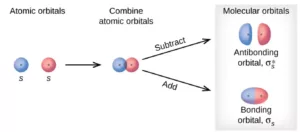Conditions for combination to atomic orbitals to form molecular orbitals:
(i) The combining atomic orbitals should have comparable energy.
(ii) The combining atomic orbitals must have the proper orientation.
(iii) The extent of overlapping should be large.

Types of Molecular Orbitals:
(i) M.Os of diatomic molecules are designated as σ (sigma), π (Pi), δ (delta), etc. the σ (sigma) M.Os are symmetrical about the bond axis, while the pi M.Os are not symmetrical.
(ii) Linear combination of two 1s atomic orbitals produces two M.Os – a BMO σ1s and an ABMO σ*1s.
(iii) Similarly linear combination of two 2s atomic orbitals produces two M.Os – σ2s and σ*2s.
(iv) If the z-axis is taken as the internuclear axis, a linear combination of two 2pz orbitals produces two sigma M.Os σ2pz and σ*2pz.
(v) While the combination of 2px and 2py orbitals produce MO’s which are not symmetrical about the bond axis. So two 2px orbitals produces 2 sigma MO’s – π2px and π*2px and two 2py orbitals produce 2 M.Os – π2py and π*2py.

Chemical Bonding and Molecular Structure

Energies of various Molecular Orbitals:
The various M.Os are filled in the increasing order of their energies (Aufbau Principle). The increasing order of energy is:
σ1s < σ*1s < σ2s < σ*2s < (π2px = π2py) <σ2pz < (π*2px= π*2py) < σ*2pz
For O2, F2 and Ne2, the order is:
σ1s < σ*1s < σ2s < σ*2s < σ2pz < (π2px = π2py) < (π*2px= π*2py) < σ*2pz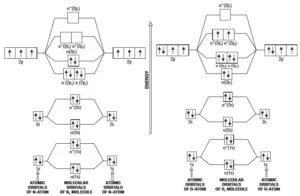Molecular Orbital Diagram for N2              Molecular Orbital Diagram for O2
Bond Order: It is defined as the half of the difference between the number of bonding electrons (Nb) and the number of anti-bonding electrons (Na).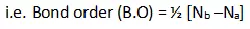A molecule is stable only if the bond order is positive. (i.e. Nb >Na). a negative bond order (i.e. Nb < Na or Nb = Na) means an unstable molecule.
For a single bond, B.O = 1, for a double bond B.O = 2, and so on. The bond order gives an approximate measure of the bond length. In general, as the bond order increases, bond length decreases, and bond enthalpy increases.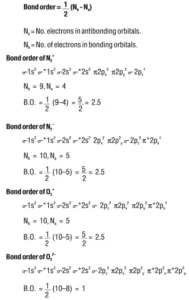Chemical Bonding and Molecular Structure

Hydrogen Bonding: The Bond which is formed between hydrogen and electronegative species such as N, O, and F. It is weaker than a covalent bond but stronger than van der Waal’s force. It is represented by the dotted line (………).
Reason for Hydrogen Bonding: When H is bonded to a strongly electronegative atom X, the shared electron pair is shifted more towards X. So, the H atom gets a slightly positive charge (δ+) and the electronegative atom gets a slight negative charge (δ). This results in the formation of a polar molecule. The electrostatic force of attraction between these polar molecules is termed H-bonding.
Thus, the hydrogen bond between the hydrogen atom bonded to the electronegative atom X may be represented as follows:
– – – X – H – – – X – H – – – X – H – – –
In such a situation, the hydrogen atom is attached simultaneously to two electronegative atoms. Hence it acts as a bridge between the two and is, therefore, called the hydrogen bridge.

Types of Hydrogen Bonding: There are two types of H bonds- intermolecular H-bonding and intramolecular H-bonding.
(i) Inter-molecular H bonding: It is the H bond formed by the H atom of one molecule and the electronegative atom of another molecule.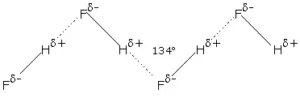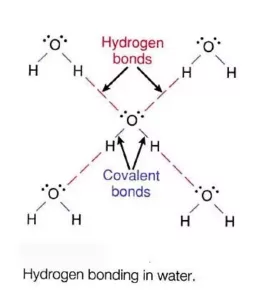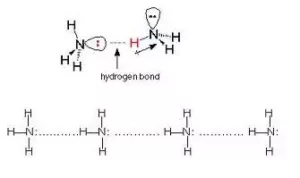Intermolecular H bonding influences the physical properties of the compounds. For example, water (H2O) is a liquid with a high boiling point but hydrogen sulphide (H2S) is a gas. This is because in water intermolecular H bonding is possible which is not possible in H2S.

(ii) Intra-molecular Hydrogen Bonding: It is the H bond formed between the H atom and the electronegative atom of the same molecule.
An important example of intramolecular hydrogen bonding is that of o–nitrophenol. The boiling point of o–nitrophenol is 214 0C as compared to 279 0C for p–nitrophenol. Further o–nitrophenol is more volatile in steam and less soluble in water than the other two isomers. All the above facts can be explained on the assumption that o–nitrophenol contains hydrogen bond represented as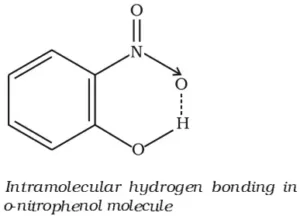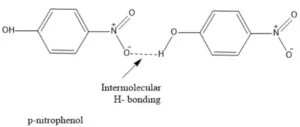Due to the intramolecular hydrogen bonding, the solubility of o–nitrophenol in water is also reduced. In m– and p– isomers, intramolecular hydrogen bonding is not possible so intermolecular hydrogen bonding takes place. This explains higher boiling points of m– and p–isomers.
Conditions for Hydrogen Bonding: In the case of intermolecular hydrogen bonding, the most important condition is that the molecules must contain one hydrogen atom linked to one highly electronegative atom. In the case of intramolecular hydrogen bonding, the following conditions are favorable for hydrogen bonding:
(i) The molecule should contain two groups such that one group contains an H–atom linked to a high electronegative atom and the other group should also contain a highly electronegative atom linked to a lesser electronegative atom.
(ii) The molecule should be planar.

Importance of Hydrogen Bond:
(i) Hydrogen Bonds are important in fixing properties such as solubilities, melting points, and boiling points and in determining the form and stability of crystalline structures.
(ii) Hydrogen bonds are important in biological systems. Proteins, for example, contain both CO and NH groups and hydrogen bonds can be formed to bridge the space between H and O. The structure and hence the properties of proteins depend upon the existence of hydrogen bonds.
(iii) Hydrogen bonding within a single molecule is one of the chief factors in determining the structure of important biological substances.

Chemical Bonding and Molecular Structure